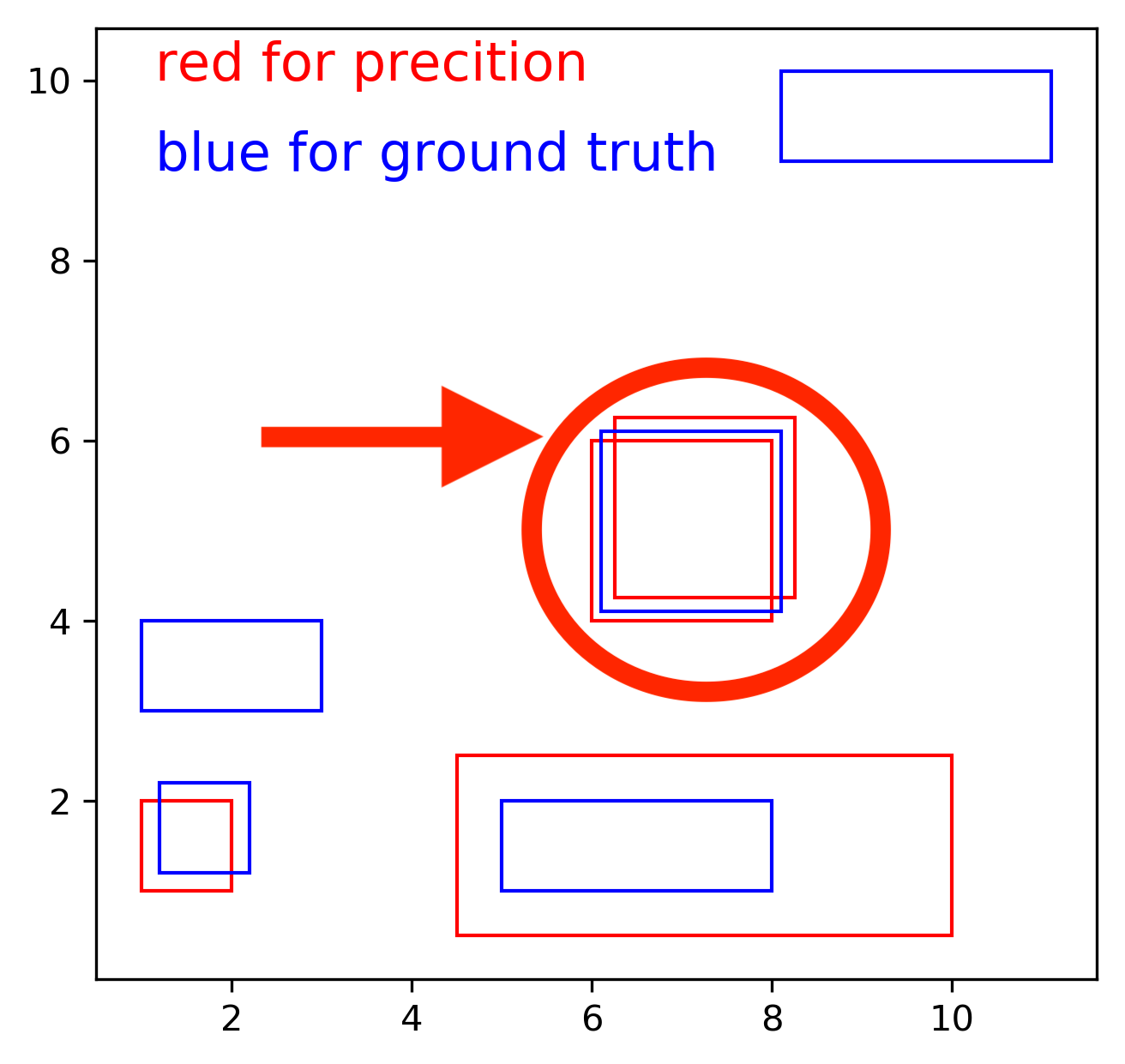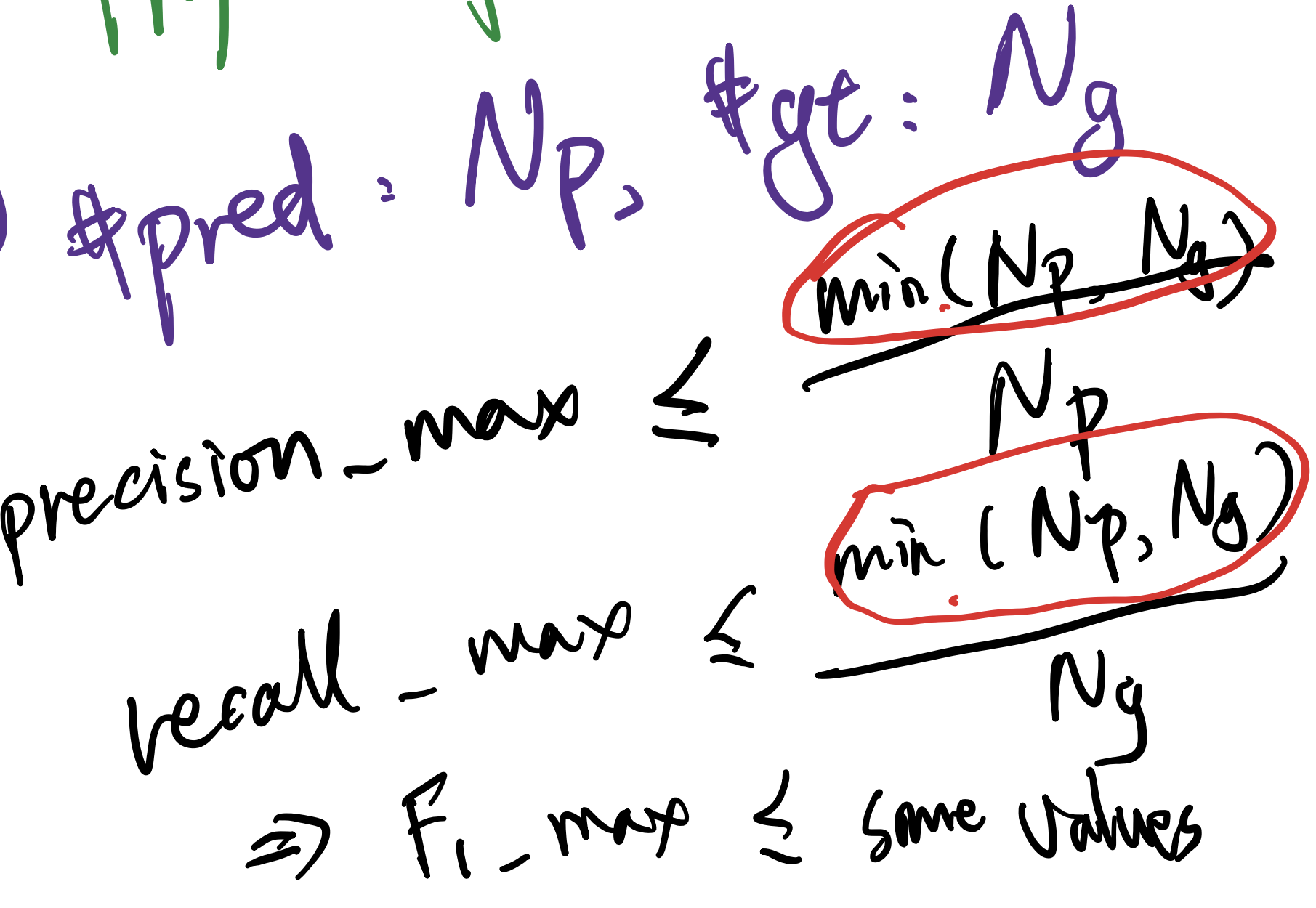# Bounding Box 匹配问题探讨

Bounding Box 是常用的 Object Detection 考虑对象而且被越来越多的用在各个学科上。但是，今天在研究的时候发现其实还有一个很细节的小问题是很容易忽略的，这就是如何匹配 Bounding Box 的问题。在这个问题 Computer Vision 和 Physics 的理解有细微的区别，这里记录下。

# 问题解释


import numpy as np

# create array data

predict = np.array([[1,2,2,1],
[4.5,2.5,10,0.5],
[6,6,8,4],
[6.26,6.26,8.26,4.26]],np.double)

truth = np.array([[1,4,3,3],
[1.2,2.2,2.2,1.2],
[5,2,8,1],
[6.1,6.1,8.1,4.1],
[8.1,8.1,11.1,9.1]], np.double)
# Below is to show the layout of the problem
# red represents truth
# blue represents prediction

import matplotlib.pyplot as plt
import matplotlib.patches as patches
fig = plt.figure()
recList = list()

# Adding red rectangle from predict
for rect in predict:
recList.append(
patches.Rectangle(
(rect, rect),
np.abs(rect - rect),
np.abs(rect - rect),
fill=False,
edgecolor = "red"
)
)

# Adding blue rectangle for truth
for rect in truth:
recList.append(
patches.Rectangle(
(rect, rect),
np.abs(rect - rect),
np.abs(rect - rect),
fill=False,
edgecolor = "blue"
)
)

# plot the graph
for p in recList:

plt.text(1.15, 10, "red for precition", size=15, color="red" )
plt.text(1.15, 9, "blue for ground truth", size=15, color="blue" )

plt.plot()
plt.show()
fig.savefig('rect2.png', dpi=300, bbox_inches='tight')non maximum suppression推荐看这个链接。 Andrew Ng 有很好的解释。

# Physical Meaningful Pairing

1. 构建 IoU Performance Matrix，其中每一个 行 代表 pred，列 代表 gt
2. 从 IoU Performance Matrix 中最大的 value 开始匹配，每匹配成功一个 value，这代表这个 value 大于我们设定的 IoU_theshold，就记录相应的匹配，同时将该 value 对应的 行 和 列 都设置为 0，这代表他们永远不可能再被使用，因为 IoU 的最小值就是 0，代表没有任何交集。

# boxA 存储的是边界框的左上顶点坐标和右下顶点坐标
# boxA=[x1,y1,x2,y2]
def iou(boxA, boxB):
# 计算重合部分的上下左右4个边的值，注意最大最小函数的使用
left_max = max(boxA,boxB)
top_max = max(boxA,boxB)
right_min =  min(boxA, boxB)
bottom_min = min(boxA, boxB)

# 计算重合部分的面积
inter = max(0,(right_min-left_max)) * max(0, (bottom_min-top_max)) # 宽*高
Sa = (boxA-boxA)*(boxA-boxA)
Sb = (boxB-boxB)*(boxB-boxB)

# 计算所有区域的面积并计算 iou
union = Sa+Sb-inter
iou = inter/union
return iou



import numpy as np

# create array data

predict = np.array([[1,2,2,1],
[4.5,2.5,10,0.5],
[6,6,8,4],
[6.26,6.26,8.26,4.26]],np.double)

truth = np.array([[1,4,3,3],
[1.2,2.2,2.2,1.2],
[5,2,8,1],
[6.1,6.1,8.1,4.1],
[8.1,8.1,11.1,9.1]], np.double)
# get useful variables
nums_pred = len(predict)
nums_gt = len(truth)

iou_matrix = np.zeros((nums_pred,nums_gt))

# boxA 存储的是边界框的左上顶点坐标和右下顶点坐标
# boxA=[x1,y1,x2,y2]
def iou(boxA, boxB):
# 计算重合部分的上下左右4个边的值，注意最大最小函数的使用
left_max = max(boxA,boxB)
top_max = max(boxA,boxB)
right_min =  min(boxA, boxB)
bottom_min = min(boxA, boxB)

# 计算重合部分的面积
inter = max(0,(right_min-left_max)) * max(0, (bottom_min-top_max)) # 宽*高
Sa = (boxA-boxA)*(boxA-boxA)
Sb = (boxB-boxB)*(boxB-boxB)

# 计算所有区域的面积并计算 iou
union = Sa+Sb-inter
iou = inter/union
return iou

def transformBBox(boxA):
# 将 BBox 从左下 + 右上 表示转换为 左上 + 右下
return [boxA, boxA, boxA, boxA]
# get iou matrix

for i in range(nums_pred):
for j in range(nums_gt):
#print(truth[j])
iou_matrix[i][j] = iou(transformBBox(predict[i]), transformBBox(truth[j]))

print(iou_matrix)

res  = []
IOU_theta = 0.4

while np.any(iou_matrix > IOU_theta):
ind = np.argmax(iou_matrix)
ind_col = ind % nums_gt
ind_row = (ind - ind_col) // nums_gt
print("row = %d, col = %d"%(ind_row, ind_col))
# store results for more analysis
res.append([predict[ind_row], truth[ind_col]])
# set the correspoding row and col to zero
# exclude those already paired from future comparsion
iou_matrix[ind_row][:] = 0
# set col to 0
for ii in range(nums_pred):
iou_matrix[ii][ind_col] = 0
print(iou_matrix)
print(res)# 总结

Written on October 23, 2020